﻿ A Comparative Performance of Conventional Methods for Estimating Market Risk Using Value at RiskPublications are Open
Access in this journal
Article Versions
Export Article
• Normal Style
• MLA Style
• APA Style
• Chicago Style
Research Article
Open Access Peer-reviewed

A Comparative Performance of Conventional Methods for Estimating Market Risk Using Value at Risk

Cyprian Ondieki Omari
International Journal of Econometrics and Financial Management. 2017, 5(2), 22-32. DOI: 10.12691/ijefm-5-2-1
Published online: April 26, 2017

Abstract

This paper presents a comparative evaluation of the predictive performance of conventional univariate VaR models including unconditional normal distribution model, exponentially weighted moving average (EWMA/RiskMetrics), Historical Simulation, Filtered Historical Simulation, GARCH-normal and GARCH Students t models in terms of their forecasting accuracy. The paper empirically determines the extent to which the aforementioned methods are reliable in estimating one-day ahead Value at Risk (VaR). The analysis is based on daily closing prices of the USD/KES exchange rates over the period starting January 03, 2003 to December 31, 2016. In order to assess the performance of the models, the rolling window of approximately four years (n=1000 days) is used for backtesting purposes. The backtesting analysis covers the sub-period from November 2008 to December 2016, consequently including the most volatile periods of the Kenyan shilling and the historical all-time high in September 2015. The empirical results demonstrate that GJR-GARCH-t approach and Filtered Historical Simulation method with GARCH volatility specification perform competitively accurate in estimating VaR forecasts for both standard and more extreme quantiles thereby generally out-performing all the other models under consideration.

1. Introduction

Over the last two decades, financial risk management has continued to be of great importance to key stakeholders in the financial industry. Value-at-Risk (VaR) is a commonly applied risk measure in finance and has become the standard tool that financial institutions and financial analysts use to quantify market risk for both internal financial risk management purposes and regulatory economic capital allocations. It is defined as the worst expected loss due to change in the value of an asset or a portfolio of financial assets under normal market conditions at a given level of probability over a specific time horizon. According to Jorion 19, VaR measures the worst expected loss over a given horizon under normal market conditions at a given level of confidence. For example, a bank might declare that the daily VaR of its trading portfolio is KES 1 million at the 99 percent confidence level. This implies that, under normal market conditions, the daily loss will exceed KES 1 million only one percent of the time.

VaR measures have many applications in the financial industry. Regulators all over the world encourage financial institutions to adopt internally based models and estimate the required capital charge based on VaR methodologies. In particular, BCBS 4 of the Bank for International Settlement (BIS) imposes requirements on financial institutions such as banks and investment firms to meet capital requirements based on the VaR estimated through internal model approach and set aside a minimum amount of capital to cover potential losses from their exposure to credit risk, operational risk and market risk. Under this approach, regulators do not provide any specific VaR measurement technique to their supervised banks – the banks are free to use their own internal based model. However, to eliminate the possible sluggishness of supervised banks to underestimate VaR so as to reduce the capital requirements, BIS has set a certain minimum standard of VaR estimates and also certain tests, such as backtesting of VaR models. If the VaR model of a bank fails in backtesting, a penalty is imposed resulting to higher capital charge. Thus, providing accurate estimates of VaR is of crucial importance for all stakeholders in the financial industry. If the underlying risk is not properly estimated, it may lead to a sub-optimal capital allocation with consequences on the profitability or the financial stability of the institutions. Financial institutions like banks are likely to consider a model that generate the lowest VaR but pass through the backtesting procedures.

The VaR’s popularity can be attributed to its theoretical and computational simplicity, its flexibility and its ability to summarize in a single value the possible losses a financial institution or an investor can lose with a given level of probability, over a given time horizon. In view of the fact that VaR is the adopted VaR measure by the Basel Committee on Banking Supervision (BCBS), a growing body of literature has either proposed a new model for measuring VaR or compares the precision of VaR estimation by the competitive models. This paper contributes to the comparison of several VaR models using a comprehensive range of parametric, non-parametric and semi-parametric models.

The conventional models of estimating VaR can generally be classified into three main categories: parametric, non-parametric and semi-parametric models. The parametric models are based on the assumption that the distribution of returns (losses) follows some specific distribution and the volatility dynamics assume a known distribution function. In order for the parametric approaches to work, one needs to explicitly specify the statistical distribution of the underlying loss data, which in turn will produce the estimates required based on their statistical properties. The non-parametric models are based on the underlying assumption that the near future will be sufficiently similar to the recent past in order to utilize the data from the recent past to forecast the risk in the near future. Non-parametric models seek to measure asset or portfolio VaR without making any limiting assumptions concerning the distribution of returns. Instead, it depends on the empirical distribution of returns to compute the tail quantiles, but cannot adequately account for the volatility clustering. Semi-parametric methods combine both parametric approaches with the non-parametric approach. Each of these techniques has its own strengths and weaknesses, but when considered together they often offer a more comprehensive point of view of financial risk measurement.

From a statistical point of view, VaR estimation entails the estimation of a quantile of the distribution of returns over the time horizon. The main complexity lies in modeling the distribution of returns, which is generally not the normal distribution. Various models for calculating VaR differ in the methodology they use and the assumptions they make about the distribution of returns. Engle and Manganelli 14 noted that the main difference among VaR models is the way they address the problem of estimating the distribution function of returns of an asset or portfolio. This is a challenge since the distribution of returns is not constant over time and given that VaR is a specific quantile of future return values subject to current information we must find an appropriate model for time-varying conditional quantiles. Many VaR models try to integrate one or more of the stylized facts of financial time series data.

In this paper, we perform an evaluation of the predictive performance of the conventional models in estimating VaR such as parametric and non-parametric methods as well as semi-parametric methods and in particular, the accuracy of the VaR model forecasts. The performance of the alternative VaR methods is assessed by using backtesting for their out-of-sample predictive ability using Kupiec’s 21 unconditional coverage tests and Christoffersen’s 11 likelihood ratio tests for coverage probability. The data set consists of daily returns of the USD/KES exchange rates over the period starting January 03, 2003 to December 31, 2016.

The rest of the paper is organized as follows. Section 2 describes the competing VaR-based approaches considered in this paper. Section 3 presents the backtesting procedures employed to perform the comparison of the VaR models. Section 4 presents the empirical results. Section 4.1 presents the descriptive statistics of the data and model parameter estimates. Section 4.2 presents the backtesting results on the relative performance of competing VaR methods based on dynamic backtesting tests for various quantile estimators. Statistical analysis of the model performance is given in Section 4.3. Finally, Section 5 contains concludes the study.

2. Value at Risk Estimation Models

Value-at-Risk (VaR) has been adopted by practitioners and regulators as the standard method of measurement of the market risk of financial assets. It summarizes in a single quantity the potential market value loss of a financial asset over a time horizon h, at a significance or coverage level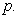Alternatively, it can be defined as a quantile of the distribution of returns (or losses) of asset or portfolio in question. Some practitioners prefer to take into consideration the negative of this quantile so that higher values of VaR correspond to a higher level of risk.

Let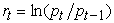be the daily negative log returns of a financial asset or portfolio, where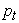is the price of an asset (or portfolio) observed at time t which can be described as follows: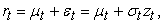(1)

where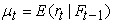is the conditional mean,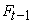is the available information until time t-1,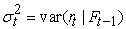is the conditional variance of the return process and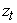is an independently and identically distributed (iid), with zero mean and unit variance, commonly referred to as white noise process.

The daily VaR that reflects the asset’s market value loss over the time horizon h, that is not expected to be exceeded with probability 1-p,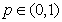, for the period t+1 denoted as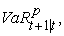is expressed as: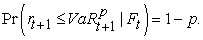(2)

where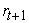is the asset’s return over the period t+1 and hence VaR is the p-th quantile of the conditional returns distribution defined as: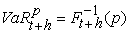(3)

where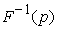is the corresponding quantile of the asset return loss distribution. For the returns process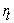, the next day’s VaR is given by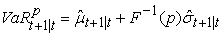(4)

where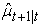and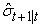are the one day ahead conditional mean and conditional volatility forecasts respectively.

This section presents the various approaches to calculating VaR examined in this paper. Section 2.1 introduces the variance-covariance method and Section 2.2 describes RiskMetrics method both parametric approaches based on the distribution of returns. Section 2.3 describes Historical Simulation method which is a non-parametric method based on the empirical distribution of past returns. Section 2.4 describes Filtered Historical Simulation method a semi-parametric approach. Finally, Section 2.5 introduces the GARCH volatility models.

2.1. Variance-Covariance Method

The Variance-Covariance method is one of the commonly used conventional approaches among the various models used to estimate VaR. The model assumes that the probability distribution of asset returns belongs to a known parametric distribution. When asset returns are assumed to be normally distributed, i.e.,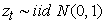for a given a data sample of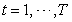daily returns, tomorrow’s VaR is computed as: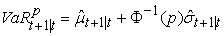(5)

where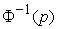is the inverse of the cumulative distribution function of the standard normal distribution while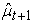and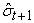are the conditional mean and volatility forecasts respectively that can be estimated by the sample mean and sample variance given by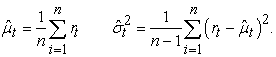(6)

Due to its simplicity, the normality assumption is widely adopted by practitioners for risk management purposes. For high quantile of a fat-tailed empirical distribution, the variance-covariance method underestimates risk since the normality assumption for financial series is usually rejected. In addition, this method is not appropriate for asymmetric distributions. This is because most financial time series exhibit nonnormal characteristics such as volatility clustering, leptokurtosis, heavy tails and the leverage effect. However, this approach has been widely applied for calculating the VaR since the risk is additive when it is based on sample variance assuming normality.

2.2. RiskMetrics

The RiskMetrics methodology developed by J. P. Morgan 25 assumes that continuously compounded return of a portfolio and/or the innovations follow a conditional normal distribution function. The basic structure of the RiskMetrics model is a restricted case of the generalized autoregressive conditional heteroscedasticity (GARCH (1, 1)) model that eliminates the need for estimating coefficients. Under the RiskMetrics approach, the conditional variance for day t+1 is computed using an Exponentially Weighted Moving Average (EWMA) which corresponds to an integrated GARCH model given by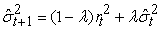(7)

whereis the return on the portfolio in period t,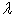is a decay factor, set to 0.94 for daily data (0.97 for monthly) in the original formulation by Riskmetrics 25. The EWMA assigns unequal weights,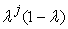on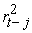particularly exponentially decreasing weights. The most recent returns are assigned more weight because they influence “today's” return more than returns further away in the past. The VaR is derived from the p-quantile of a normal distribution: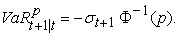(8)

The main advantage of this method is that it allows for a complete specification of the distribution of returns and there may be room for improving the performance by avoiding the normality assumption. RiskMetrics approach generally tends to underestimate the VaR, because the normality assumption of the standardized residuals seems not to be consistent with the characteristics of financial returns. Empirical evidence confirms that financial returns do not follow the normal distribution. The skewness coefficient is in most cases statistically significant negative value, implying that the financial return distribution is skewed to the left which is not in agreement with the properties of a normal distribution. Also, empirical distribution of financial return exhibit significantly fat tails and excess kurtosis according to Bollerslev 7. One of the challenges of RiskMetrics is that EWMA model captures some non-linear characteristics of volatility, such as varying and clustering volatility but does not take into account asymmetry and the leverage effect.

2.3. Historical Simulation

Historical Simulation (HS) was introduced by Boudoukh et al 8 and Barone Adesi 2 as a method for estimating Value-at-Risk. HS is a nonparametric method that is widely used due to its simplicity in implementation and ease of interpretation to different players in the financial industry. According to Perignon and Smith 24 of the 64.9% of firms that disclosed their VaR estimation methodology, 73% reported using Historical Simulation rather than the parametric linear or Monte Carlo VaR methodologies. The HS approach to calculating VaR assumes that all possible future variations have been experienced in the past and that the historically simulated distribution is identical to the returns distribution over the forward-looking risk horizon. The HS approach simplifies the procedure for computing the VaR since it doesn’t make any distributional assumption about portfolio returns. Therefore, the VaR is estimated as: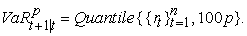(9)

HS has the advantage over more sophisticated models since it captures the fat-tailed and other non-normal characteristics of financial asset returns without knowing the underlying distribution. However, it also has some weaknesses. First, the VaR estimates are completely dependent on the selected historical data and ignore any other events that are not captured in the data. For volatile periods, it can be a very bad measure of risk since it will often overestimate risk and during calm periods the method will often underestimate risk. VaR estimates based on the HS methodology are biased downwards (correspondingly upwards) since it will take some time to reflect major events; such as the increases in risk associated with sudden market turbulence. It is also problematic to use the HS approach to forecasting out-of-sample VaR when the distribution over the sample period does not represent the population distribution. Finally, VaR estimates based on historical simulation may present predictable jumps, due to the discreteness of extreme return. A more comprehensive discussion on the use of Historical Simulation approach for VaR estimation can be found in various articles such as Hendricks 17 and Barone-Adesi et al. 3.

2.4. Filtered Historical Simulation

Filtered Historical Simulation (FHS) method is a semi-parametric method proposed by Hull and White 18 and Barone-Adesi et al. 2 that combines the power and flexibility of parametric approach and the benefits of non-parametric method HS. FHS is an alternative to traditional HS technique and Monte Carlo (MC) simulation approach. Filtered Historical Simulation incorporates a nonparametric characteristic of the probability distribution of asset returns with a relatively complex model-based treatment of volatility (e.g. GARCH). Assuming that asset returns are i.i.d., future VaR can be well approximated by the empirical distribution of historical returns. In this case, the VaR is calculated as the p-th empirical quantile of the unconditional return distribution of a moving window of w historical observations 10.

To use FHS to estimate VaR of a financial asset over a 1-day horizon, the first step in applying this technique is to fit a conditional volatility model to the asset return data. Barone-Adesi et al. 2 suggest an asymmetric GARCH model. The realized returns are then standardized by splitting each by the corresponding volatility,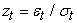. These standardized returns should be suitable for HS. The third step consists of bootstrapping a large number of drawings from the sample set of standardized returns. By using the quantiles of the standardized residuals, the conditional standard deviation and the conditional mean forecasts from a volatility model, the VaR estimate is given as: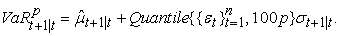(10)

Barone-Adesi and Giannopoulos 3 proved that the FHS risk measure is superior to the Historical Simulation since it generated better VaR forecasts that are consistent with the current state of markets at any arbitrarily large confidence level. As a result, this method accommodates volatility clustering, leptokurtosis and other factors that have an asymmetric effect on the volatility of the empirical distribution.

2.5. GARCH Models

The GARCH models are used to account for the time- varying nature of the mean and variance of returns. Engle 13 proposed the Autoregressive Conditional Heteroscedastic (ARCH) model which features the conditional variance modeled as a linear function of past squared innovations that does not remain fixed but rather varies throughout the period. Bollerslev 6 extended the ARCH model to the Generalized Autoregressive Conditional Heteroscedastic (GARCH) model. The GARCH model is generalized in the sense that the squared conditional volatility is a linear function of past squared conditional volatilities and squared innovations of the process. The GARCH model specification has two main components: the mean component that captures the evolution of returns in accordance with earlier returns and the variance component that formulates the evolving volatility of returns. The standardized residuals are assumed to be independently and identically distributed (iid). The generalized formulation for the GARCH (p, q) model is given by: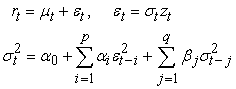(11)

wheredenotes returns at time t,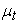is the conditional mean, andis a sequence of independently identically distributed random variables with zero mean and unit variance.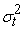is the conditional variance of innovations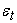given information at time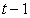and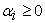, for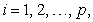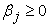for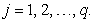The value of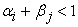is known as the model's persistence, which reflects how long a shock to the long-run average variance will persist. The parameter restrictions are necessary to ensure positive conditional variance as well as covariance stationary. The GARCH (1, 1) model is the simplest and has been successfully applied to financial data. The empirical applications indicate that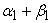is always observed to be very close to the unit.

The one-step-ahead conditional variance forecast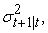for the GARCH (p, q) model is given by: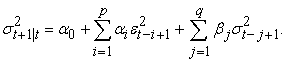(12)

The ARCH and GARCH models are both symmetric; that is, the sign of the innovations or shocks have no effect on the conditional volatility, only the squared innovations enter the conditional variance equation. This is, however, inconsistent with the stylized fact that negative shocks (bad news) tend to have a larger impact on volatility than the positive shock (good news) of the same magnitude (leverage effect) according to Black 5.

The Exponential GARCH (EGARCH) model is an extension of the GARCH model introduced by Nelson 23 that overcomes the weaknesses of the GARCH model. The EGARCH model takes into consideration the leverage effects and does not impose any parameter restrictions on the model. It allows positive and negative shocks to have a different impact on volatility and also large shocks to have a greater impact on volatility. The conditional variance equation of EGARCH (p, q) model is given by: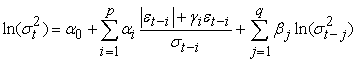(13)

The one-step-ahead conditional variance forecast for the EGARCH (p, q) model is given by: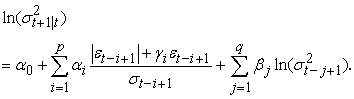(14)

The GJR-GARCH model is an asymmetric GARCH model, proposed by Glosten et al. 16. The GJR-GARCH model also captures other stylized facts of financial time series like volatility clustering. The variance equation of GJR-GARCH (p, q) is given by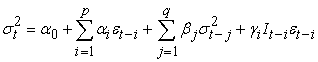(15)

where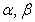and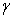are constant parameters, and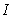is an indicator function (dummy variable) that takes the value zero when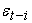is positive and one whenis negative. If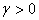, negative innovations or bad news has a greater impact than positive innovations or good news. The parameters of the model are assumed to be positive and that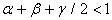. When all the leverage coefficients are zero, the GJR-GARCH model reduces to the standard GARCH model.

To specify GARCH models, it is necessary to assume the conditional distribution of the innovations term and the most commonly used distributions include: normal, Student’s t and generalized error. For a given distribution, the parameters of the GARCH model are estimated by the pseudo-maximum likelihood (PML) method. The log likelihood function of the GARCH (1, 1) model, with T independently and identically distributed observations, assuming normal distribution of the innovations is given by: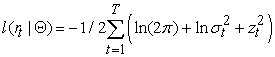(16)

where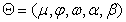is the parameter vector of the AR-GARCH(1,1) model. In this case, VaR can be computed as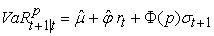(17)

where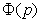denotes the percentile at p for standard normal distribution. According to Bollerslev 7, using standardized Student’s t distribution as the conditional distribution for the stochastic component of the GARCH process can be considered acceptable, since it shows thicker tail and excess kurtosis than the normal distribution. Under this assumption, VaR can be computed as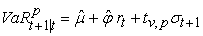(18)

where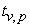denote the percentile at p for standard Student-t distribution with v degrees of freedom.

3. Statistical Backtesting Methods

Backtesting is a set of statistical procedures designed to check whether the actual losses are in line with VaR forecasts 20. It provides an invaluable feedback about the accuracy of the models to risk managers and the users of VaR. A number of different backtesting procedures have been proposed in the statistical literature and most of these rely on applying quantitative methods for testing the consistency between the model-estimated risk measures and the assumptions of the tested risk model 12. Regulators require that the institutions’ internal risk models are validated on both a 97.5% and 99% confidence level, for a minimum period of one year, although additional backtesting should also be considered like using different confidence levels as well as longer time periods 4.

The backtesting of VaR estimates is performed based on Kupiec’s 21 unconditional coverage test and conditional coverage test proposed by Christoffersen 11. The unconditional coverage test is used to determine whether the frequency of failures in the sample is in line with the expected number of violations of the predicted VaR models. Although the unconditional coverage is accurately evaluated, the inherent variance dynamics are overlooked and the conditional coverage at specific points in time is ignored. Hence, an otherwise inadequate VaR model could be accepted. The conditional coverage test is a joint test that considers both correct unconditional coverage and serial independence of the failure process.

3.1. Unconditional Coverage Backtesting

The unconditional coverage (UC) test also known as the POF-test (proportion of failures) tests if the proportion of failures, i.e. the actual number of VaR violations over a given time period equals to the expected allowable number of VaR violations. Let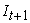be the indicator sequence of VaR violations that can be described as: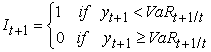(19)

and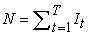is the number of days over a T period that the portfolio loss was greater than the VaR forecast. The null hypothesis of Kupiec’s unconditional coverage test assumes that the probability of occurrence of the violations, N/T equals the expected significance level p. Under the null hypothesis of the model being ‘correct’, the number of exceptions follows the binomial distribution and, consequently, the appropriate likelihood ratio statistic is given by: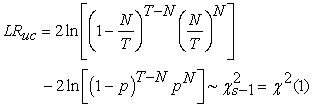(20)

where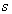is the number of possible outcomes and the UC test, therefore, follows an asymptotically Chi-squared distribution with one degree of freedom. The null hypothesis is rejected if the VaR model generates too few or too many violations. The Kupiec test only provides a necessary condition to classify a VaR model as adequate but it does not account for the possibility of clustering of violations, which can be caused by volatile return series. Thus, backtesting VaR model should not rely exclusively on tests of unconditional coverage, 9. VaR model validation is also reliant on a test of the randomness of these VaR violations, to avoid clustered violations.

3.2. Conditional Coverage Backtesting

An accurate VaR model must exhibit both the unconditional coverage and independence property. Accordingly, tests that jointly examine the unconditional coverage and independence properties provide an opportunity to detect VaR measures which are deficient in one way or the other. 9. Christoffersen 11 proposed a conditional coverage test that jointly investigates (i) whether the number of VaR violations is statistically consistent with the allowable proportion of VaR violations (unconditional coverage) (ii) whether the VaR violations are independently distributed through time, i.e., no clustering of violations, and (iii) whether there is clustering with the proportion of VaR violations within allowable limits (conditional coverage).

Under the null hypothesis that the violations are independently distributed through time and the failure rate is equal to the expected proportion of violations p, the appropriate likelihood ratio statistic for the conditional coverage test is: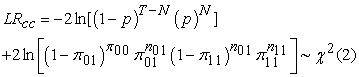(21)

Where nij is the number of observations with value i followed by j for i, j = 0, 1 and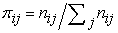are the corresponding probabilities. i, j = 1 indicate that a violation has been made, while i, j = 0 indicates the opposite. The advantage of the conditional coverage test is that it can reject those VaR models that generate either too many or too few clustered violations, but it requires a number of observations to become more accurate. Rejection of the null hypothesis leads to the conclusion that the model is not adequate in maintaining allowable VaR violations and is vulnerable to VaR exceedance clustering. Non-rejection of this hypothesis leads to be confident in the reliability of the VaR model in the aspect of predicting events of losses.

4. Empirical Results

4.1. Data and Descriptive Statistics

The data consists of the daily average exchange rate of the USD/KES for the period from January 3, 2003, to December 31, 2016. The data consists of 3050 observations of daily returns. The continuously compounded daily returns are defined by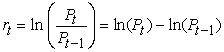(22)

whereis the daily exchange rate return and Pt and Pt-1 denote the average daily exchange rate of the USD/ KES at the current day and previous day, respectively.

Figure 1 presents daily currency exchange prices trends corresponding to the analyzed period and indicates that the currency prices have demonstrated some characteristics of financial time series data. The currency prices data exhibit volatility clustering in some time periods, as upward movements tend to be followed by other upward movements and downward movements also followed by other downward. The daily currency exchange log-returns are computed and plotted in Figure 2 which shows large changes are followed by large changes and small changes are followed by small changes, such as in the years 2008-2009 and 2011-2012 when currency exchange returns were extremely volatile. In this period, the financial markets were characterized by unusually high volatility levels and huge losses due to the effects of the world financial crisis. Similarly, the first quarter 2011 was dominated by high volatility and large losses. The returns plot illustrates that returns are stationary and a conditional heteroscedastic model can be proposed to model the daily currency exchange price returns where large changes are followed by large changes and small changes are followed by small changes.

• Figure 1. Daily average prices series for daily exchange rates
• Figure 2. Daily log returns series for daily exchange rates

Table 1 presents summary descriptive statistics for the full sample, as well as an in-sample period of daily currency exchange returns used subsequently in model estimation. The full sample daily returns have a positive mean and are slightly skewed to the left which means there is a long tail in the negative direction. The standard deviation of daily returns exhibits high volatility. The magnitude of the average return is very small compared to the standard deviation. Further, the series exhibit significantly high excess kurtosis indicating that the distribution of returns follows a fat-tailed distribution, thereby exhibiting an important characteristic of financial time series data, namely leptokurtosis. The Jarque-Bera statistic indicates that daily returns are not normally distributed. The Ljung–Box statistic for serial correlation shows that null hypothesis of no autocorrelation is rejected for up to 20th lag at any level of significance and confirms the presence of conditional heteroskedasticity. This indicates a GARCH-type modeling should be considered in the estimation of the Value at Risk of currency exchange returns. Asymmetric GARCH models may also prove practical, because of the leverage effect. A distribution other than the normal (e.g. the Student t distribution) for the errors may be considered, because of the excess kurtosis and the fat-tailed nature of the data.

Table 2 presents the parameter estimates of the mean and volatility equations of currency exchange returns for some of the fitted GARCH models. The parameter estimates for mean and conditional volatility equations are based on the in-sample period. These models are fitted to returns series using a pseudo maximum likelihood estimation method assuming normal and student’s t distributed innovations to obtain parameter estimates and standardized residuals. The VaR models’ adequacy and efficiency were assessed based on the Akaike information criterion (AIC) and Bayesian information criterion (BIC) criteria by selecting a model specification with minimum AIC, BIC and largest log-likelihood function and significant parameter estimates. Angelidis et al. 1 pointed out that the conditional mean specification in the GARCH(1, 1) process does not seem to influence significantly the accuracy of the VaR estimates. The AR(1)-GARCH(1, 1) model specification with both normal and Student’s t distributions for the innovations was assumed to be adequate on each rolling window. Both the constant terms and autoregressive AR (1) coefficients in the mean equations are found to be significant, except constant term of the mean equation under normal innovations. Similarly, most of the parameters in the volatility equations: the constant, the ARCH(1) parameter and the GARCH (1, 1) parameter, are significant except for the EGARCH model.

The AR(1)-GARCH(1, 1)-normal model is used in two contexts. In the first context, the model is used directly as a risk measurement methodology for comparison with other conventional risk measurement methods. Secondly, the model is used to pre-filter the return series and generate the standardized residuals which are inferred by the Filtered Historical Simulation approach. The AR (1)-GARCH (1, 1) specification is estimated using a rolling window of 1000 days data.

The standardized residuals extracted from the estimated model are utilized in two ways: (i). to investigate the adequacy of ARCH modeling, and (ii), to be used in the FSH approach. Figure 3 illustrates the results of filtering the return series data with a GARCH modeling framework. The i.i.d. assumption may be reasonable for residuals. This shows that AR (1)-GARCH (1,1) model sufficiently explains the heteroscedasticity effect in the returns, thus we can conclude that the model fit the USD/KES returns well.

• Figure 3. Correlograms for the raw data and their absolute values as well as for the residuals and absolute residuals

Table 3 reports the results of testing Normality (Jarque–Bera), autocorrelation (Ljung-Box) and ARCH effects (LM-Test), for the return series data as well as for the standardized residuals of currency exchange returns. The Ljung-Box test with 20 lags applied to the return series data produces evidence of serial correlation. The results also indicate that the return series data exhibit excess kurtosis and significant ARCH effects. The residual series are also found to have significant excess kurtosis but it doesn’t possess any significant serial autocorrelation or any ARCH effect left suggesting that the functional form is adequate for the data. The results can be summarized that neither the return series nor residual series can be considered to be normally distributed since both series have significant excess kurtosis. Therefore, the assumption of conditional normality is impractical. On the other hand, the residual series are found to be free from any serial autocorrelation and hence we can conclude that the filtering process successfully eliminates the time series dynamics from the return series and an i.i.d. series free from any time series dynamics is obtained.

4.2. Dynamic Backtesting

The VaR models are backtested using the Kupiec 21 test for unconditional coverage and the Christoffersen 11 test for conditional coverage probability. In order to analyse VaR model accuracy, five confidence levels of 95%, 97.5%, 99%, 99.5% and 99.9% are considered to reflect the extreme market conditions. The evaluation is based on one-step-ahead forecasts that have been produced from a series of rolling samples size with an estimation window of 1000 observations kept constant and simply rolled forward one day at a time. The procedure is repeated for the remainder of the daily observations in the sample data. The last eight years of the sample data are preserved for performance evaluation purposes of the VaR models, hence T=2000 days.

The rolling window technique eliminates 8 overlapping data in the test sample and captures the dynamic time-varying characteristics of the return series data in different time periods. McNeil and Frey 22 and Gençay et al. 15, acknowledged that within the backtest period, it is practically impossible to examine the fitted model carefully every day and to choose the best parameterization.

The evaluation of the overall performance of the models during the backtesting period takes into consideration the number of violations of the null hypothesis for each VaR model. The relative out-of-sample performance of each VaR model is evaluated by comparing the actual and expected violation ratios and competing models are ranked accordingly by the absolute deviation from the expected violation ratios. A violation occurs if the realized empirical next-day return is greater than the forecasted one on a particular day. The violation ratio is defined as the total number of times that the realized return is larger than the estimated return (number of violations), divided by the total number of one-period forecasts. When forecasting VaR estimates at a certain quantile q, we expect the realized return will be higher 100(1-q) percent of the time if the model is correct. Generally, the violation ratio should converge to q as the sample size increases. A violation ratio at the pth quantile higher than q percent implies that the model consistently underestimates the realized return/risk at the tail. On the other hand, a violation ratio less than p percent at the pth quantile indicates that the model consistently overestimates the realized return which will require excessive capital commitment. For example, if the expected VaR violation ratio is 5% at the 95th quantile, it implies that the realized return is only 5% of the time greater than what the model predicts. This approach is justified since a model that is never rejected by the POF test is certainly preferable to a rejected (one or more times) model: thus, a VaR model with fewer rejections is considered more accurate. Both the unconditional and conditional coverage tests only deal with the frequency of the violations and are used to test the statistical accuracy of the various risk management models.

Table 4 presents the summary of the relative out-of-sample performance of each VaR method in terms of the VaR violation ratio for the left tail (losses) and the right tail (gain) using a rolling window of 1000 observations. A violation ratio that is greater than the expected ratio indicates an excessive underestimation of the risk which implies that the model recommends less capital allocation while a violation ratio less than the expected ratio indicates an excessive overestimation which implies a greater capital allocation than necessary.

Table 5 presents the corresponding p-values for the ten methods implemented at five different significance levels for the unconditional coverage test. The null hypothesis of Kupiec’s 21 unconditional coverage test assumes that the probability of occurrence of the violations equals the expected significance level. A p-value greater 0.05 for the unconditional coverage test indicates that the number of violations is statistically equal to the expected one. The null hypothesis of the conditional coverage test states that the probability of occurrence of the violations equals the expected significance level and the violations are independently distributed through time. A p-value less than or equal to 0.05 for the conditional coverage test shows that the model generates too many or too few clustered violations and will be interpreted as evidence for rejecting the null hypothesis.

Table 6 presents the corresponding p-values at five different significance levels for the conditional coverage test sample performance of various methods considered. The main evidence from the backtesting procedure is that the models perform relatively well at high confidence levels (from 99% up to 99.5%).

4.3. Statistical Analysis of Model Performance

The performance of competing approaches to VaR measurement is based on an assessment of the out-of-sample accuracy of estimated quantiles. Specifically, the out-of-sample violation proportions are compared to theoretical probabilities. In terms of VaR performance, it is difficult to draw consistent conclusions across various methods and quantile levels. Of the parametric models, the GJR-GARCH-t method produces the most accurate forecasts of VaR amongst all the ten competing models. The model gives the most accurate forecast of risk at all quantiles and performs better for the left tail than the right tail. The success rate for the left tail is 100% as compared to the success rate of 80% for the right tail. Generally, the GARCH-t model is well suited for VaR estimation and is very successful for the right tail and for almost all confidence levels and also seems to produce equally good results for the left tail. Its overall success rate is 80% for both left and right tails. The Filtered Historical Simulation approach performs fairly in terms of forecasting accurate VaR for both tails and provides an improvement to the GARCH-normal models. Its overall success rate is 60% for both tails. The performance of the GARCH-normal models is not very good with GJR-GARCH and GARCH having an overall success rate of 50% respectively and E-GARCH having an overall success rate of 40%. The RiskMetrics provides an improvement to the historical simulation approach with an overall success rate equal to 50%. The historical simulation method and unconditional normal methods performed worst and are rejected by the two backtesting measure at all confidence levels except at (99% VaR). Most often the models underestimate the risk exposure and hence are rejected by the two backtesting measures. The overall success rate, i.e. across the five levels of significance is equal to 20% for both the historical simulation model and unconditional normal model. This is expected as the actual returns exhibit excess kurtosis and negative skewness. The results confirm the hypothesis that the widely-used normal distribution based VaR models tend to underestimate the true risk.

5. Conclusion

This paper compares the predictive performance of ten different VaR models commonly employed in market risk measurement. The various VaR models compared include; unconditional normal, Historical Simulation, Exponential Weighted Moving Average (EWMA/RiskMetrics), Filtered Historical Simulation, GARCH models under Normal distribution and Student-t distributions. The out-of-sample period is used to compare the VaR performance of the alternative models. A rolling window size of 1000 data points is used and estimations for both tails of returns distribution at five confidence levels 95%, 97.5%, 99%, 99.5% and 99.9% were considered to better reflect the extreme market conditions.

The paper also examines the performance of VaR models by assessing the unconditional and conditional interval coverage of the various approaches to forecasting VaR. Assessing conditional coverage is important if true tail quantiles are time varying. In such cases, simple VaR approaches based on Historical Simulation are likely to result in ‘clustering’ of VaR violations, and this will occur during periods of extreme events in the market when accurate VaR forecasts are required most. The statistical backtesting tests of Christoffersen 11 suggest that the HS approach does indeed fail to provide adequate conditional coverage. In contrast, the GJR-GARCH approach generates VaR forecasts that provide appropriate conditional coverage. These findings indicate that the conditional approaches are preferable to the unconditional approaches in Value-at-Risk measurement.

In summary, the GJR-GARCH model and the Filtered Historical Simulation models perform best amongst all the VaR models considered based on the two backtesting measures and in general the GARCH-Student models generally perform better than the GARCH-normal models. The models with Gaussian assumption generally underestimate VaR as they fail to capture the leptokurtosis which is often observed in financial returns. The Historical Simulation method and unconditional normal methods perform the worst for the two backtesting measures. These findings are consistent across nearly all the quantiles examined in this paper. For further research, several areas still remain open. First, we have only used a single currency exchange rate as a single asset. A more realistic “portfolios” consisting of more assets would be quite important. We suggest the modeling of portfolios with more than one asset, and secondly, the analysis of extreme events via Extreme Value Theory models can also be considered.

References

  Angelidis, T., Benos, A., & Degiannakis, S. (2004). The use of GARCH models in VaR estimation. Statistical methodology, 1(1), 105-128. In article View Article  Barone -Adesi, G. G. (1999). VaR without correlations for portfolio of derivative securities. Università della Svizzera italiana. In article View Article  Barone-Adesi, G., & Giannopoulos, K. (2001). Non parametric VaR techniques. Myths and realities. Economic Notes, 30(2), 167-181. In article View Article  Basel Committee on Banking Supervison. (2013). Fundamental review of the trading book: A revised market risk framework. In article  Black, F. (1976). Studies of stock price volatility changes. In: proceedings of the 1976 Business Meeting of the Business and Economics Statistics Section, American Association, 177-181. In article  Bollerslev, T. (1986). Generalized autoregressive conditional heteroskedasticity. Journal of econometrics, 31(3), 307-327. In article View Article  Bollerslev, T. (1987). A conditionally heteroskedastic time series model for speculative prices and rates of return. The review of economics and statistics, 542-547. In article View Article  Boudoukh J., Richardson M., Whitelaw R. (1998). The best of both worlds, Risk 11 (May), 64-67. In article View Article  Campbell, S. D. (2006). A review of backtesting and backtesting procedures. The Journal of Risk, 9(2). In article View Article  Christoffersen, P. (2009). Backtesting. Encyclopedia of Quantitative Finance. In article View Article  Christoffersen, P. F. (1998). Evaluating interval forecasts. International economic review, 841-862. In article View Article  Dowd, K. (2005). Measuring market risk. John Wiley & Sons, Ltd. In article View Article  Engle, R. F. (1982). Autoregressive conditional heteroscedasticity with estimates of the variance of United Kingdom inflation. Econometrica: Journal of the Econometric Society, 987-1007. In article View Article  Engle, R. F., & Manganelli, S. (1999). CAViaR: conditional value at risk by quantile regression (No. w7341). National bureau of economic research. In article View Article  Gencay, R., Dacorogna, M., Olsen, R., & Pictet, O. (2003). Foreign exchange trading models and market behavior. Journal of Economic Dynamics and Control, 27(6), 909-935. In article View Article  Glosten, L. R., Jagannathan, R., & Runkle, D. E. (1993). On the relation between the expected value and the volatility of the nominal excess return on stocks. The journal of finance, 48(5), 1779-1801. In article View Article  Hendricks, D. (1996). Evaluation of value-at-risk models using historical data (digest summary). Economic Policy Review Federal Reserve Bank of New York, 2(1), 39-67. In article View Article  Hull, J., & White, A. (1998). Incorporating volatility updating into the historical simulation method for value-at-risk. Journal of Risk, 1(1), 5-19. In article View Article  Jorion, P. (2001). Financial Risk Manager Handbook 2001-2002. Wiley. In article  Jorion, P. (2007). Financial risk manager handbook (Vol. 406). John Wiley & Sons. In article View Article  Kupiec, P. H. (1995). Techniques for verifying the accuracy of risk measurement models. The journal of Derivatives, 3(2), 73-84. In article View Article  McNeil, A. J., & Frey, R. (2000). Estimation of tail-related risk measures for heteroscedastic financial time series: an extreme value approach. Journal of empirical finance, 7(3), 271-300. In article View Article  Nelson, D. B. (1991). Conditional heteroskedasticity in asset returns: A new approach. Econometrica: Journal of the Econometric Society, vol.59, No.2, 347-370. In article View Article  Pérignon, C., & Smith, D. (2010). The level and quality of value-at-Risk disclosure by commercial banks, Journal of Banking and Finance 34, pp. 362-377. In article View Article  Riskmetrics, T. M. (1996). JP Morgan Technical Document. In articleThis work is licensed under a Creative Commons Attribution 4.0 International License. To view a copy of this license, visit http://creativecommons.org/licenses/by/4.0/